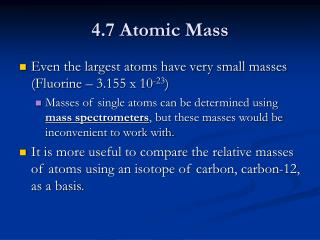Download Presentation4.7 Atomic Mass

# 4.7 Atomic Mass - PowerPoint PPT PresentationDownload Presentation## 4.7 Atomic Mass

- - - - - - - - - - - - - - - - - - - - - - - - - - - E N D - - - - - - - - - - - - - - - - - - - - - - - - - - -
##### Presentation Transcript

1. 4.7 Atomic Mass • Even the largest atoms have very small masses (Fluorine – 3.155 x 10-23) • Masses of single atoms can be determined using mass spectrometers, but these masses would be inconvenient to work with. • It is more useful to compare the relative masses of atoms using an isotope of carbon, carbon-12, as a basis.

2. 4.7 Atomic Mass • Carbon-12 was assigned a mass of exactly 12.00000 amu atomic mass unit (amu) – one-twelfth the mass of a carbon-12 atom • Since carbon-12 has a mass number of 12 (6 protons and 6 neutrons), the mass of a single proton or neutron is approximately 1 amu.

3. 4.7 Atomic Mass • Since most of the mass of an atom depends on the number of protons and neutrons in the nucleus you may expect the atomic mass to be a whole number – this is not the case.

4. 4.7 Atomic Mass • In nature, most elements occur as a mixture of two or more isotopes • Each isotope has a fixed mass and a natural percent abundance atomic mass – a weighted average mass of the atoms in a naturally occurring sample of the element • This reflects both the mass and the relative abundance of the isotopes as they occur in nature

5. 4.7 Atomic Mass • Example: Hydrogen-Atomic mass: 1.0079 amu • Three isotopes: Hydrogen-1, Hydrogen-2, Hydrogen-3 • Hydrogen-1 has a mass of 1.0078 and a natural abundance of 99.98% • Hydrogen-2 and hydrogen-3 occur in trace amounts • The slight difference in the mass of hydrogen-1 and the average atomic mass takes into account the other two isotopes.

6. 4.8 Calculating the Atomic Mass • Because the atomic mass must reflect both the masses and the relative natural abundances of the isotopes, you must know: • The number of stable isotopes of that element • The mass of each isotope • The natural percent abundance of each isotope

7. 4.8 Calculating Atomic Mass • Example: Element X has two natural isotopes. The isotope with mass 10.012 amu has a relative abundance of 19.91 %. The isotope with mass 11.009 amu has a relative abundance of 80.09%.Calculate the average atomic mass and determine what element it is.

8. 4.8 Calculating Atomic Mass • Solution: • Find the mass that each isotope contributes to the weighted average by multiplying the mass by its relative abundance. Then add the products. X 10.012 amu x 0.1991 = 1.993 amu X 11.009 amu x 0.8009 = 8.817 amu Total: 10.810 amu Element X is boron. 10 11

9. 4.8 Concept Practice 20. The element copper contains the naturally occurring isotopes C and C. The relative abundances and atomic masses are 69.2% (mass = 62.93 amu) and 30.8% (mass = 64.93 amu), respectively. Calculate the average atomic mass of copper. 63 29 65 29

10. 4.8 Concept Practice 20. Solution: 62.93 amu x 0.692 = 43.5 amu 64.93 amu x 0.308 = 20.0 amu Total: 63.5 amu